GFG App
Open AppBrowser
Continue

# How to Calculate Point-Biserial Correlation in Excel?

The Point-Biserial Correlation Coefficient is a correlation metric that measures the degree of relationship between a continuous and a binary variable. The connection between a binary variable, x, and a continuous variable, y, is measured using point-biserial correlation. Binary variables are widely used to describe the presence of a certain attribute or membership in a group of observed specimens. Create a binary variable from ordinal or continuous-level data because ordinal and continuous-level data include more variance information than nominal data and so improve the reliability of any correlation study.

## Point-Biserial Correlation Coefficient

The point-biserial correlation coefficient, like the Pearson correlation coefficient, has a value between -1 and 1 where:

• A correlation between two variables that is entirely negative is represented by the number -1.
• 0 means that there is no connection between the two variables.
• A correlation coefficient of 1 denotes a totally positive relationship between two variables.

This will demonstrate how to compute the point-biserial correlation between two variables. It only accepts two value ranges as arguments.

= CORREL ( Variable1, Variable2 )

Variables 1 and 2 are the two variables for which you wish to compute the Point-Biserial Correlation.

Example 1: Assume we have a binary variable, x, and a continuous variable, y: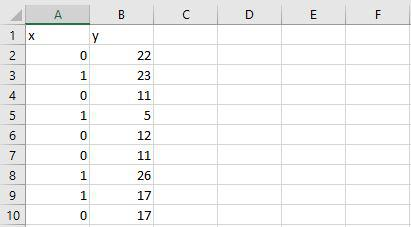We can easily use the =CORREL() method to determine the point-biserial correlation between x and y.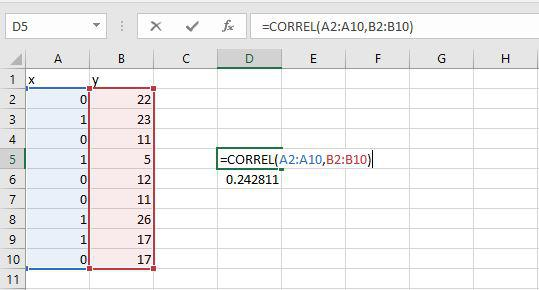The point-biserial correlation between x and y is 0.242811. Although this number is positive, it implies that when the variable x is set to “1,” the variable y tends to take on greater values than when the variable x is set to “0.” This is simply demonstrated by computing the average value of y when x is 0 and when x is 1.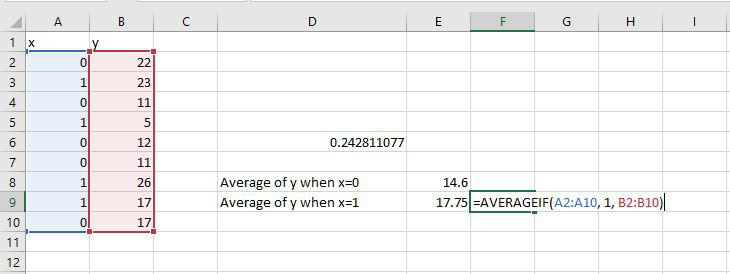The average value of y for x = 0 is 14.6. The average value of y for x = 1 is 17.75. This confirms that the two variables’ point-biserial correlation should be positive.

Example 2: Assume we have a continuous variable y, and a binary variable x: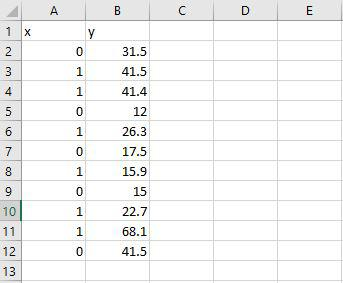We can simply find the point-biserial correlation between x and y using the =CORREL() method: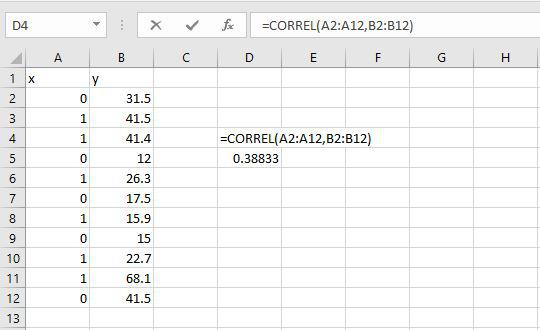The point-biserial correlation between x and y is 0.38833. Although this number is positive, it means that when variable x is set to “1,” variable y tends to take on larger values than when variable x is set to “0.” Simply compute the average value of y when x is 0 and when x is 1 to illustrate this: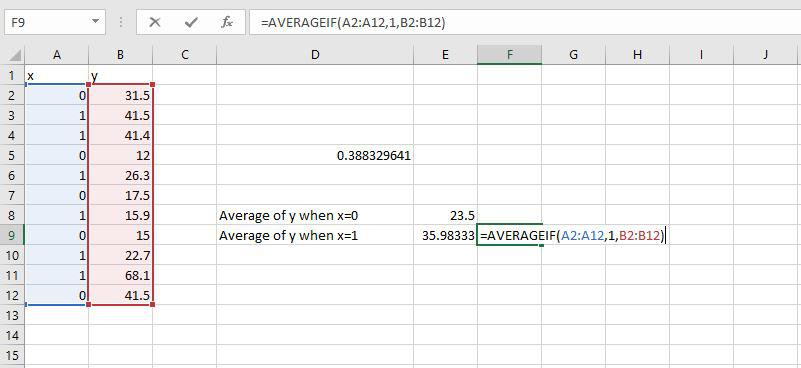For x = 0, the average value of y is 23.5. For x = 1, the average value of y is 35.983. This shows that the point-biserial correlation between the two variables should be positive.

My Personal Notes arrow_drop_up